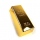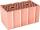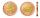Pounds

3 pounds subtract 1/3 of a pound.

Result

x =  2.667

Solution:Leave us a comment of this math problem and its solution (i.e. if it is still somewhat unclear...):Be the first to comment!To solve this verbal math problem are needed these knowledge from mathematics:

Need help calculate sum, simplify or multiply fractions? Try our fraction calculator.

Next similar math problems:

1. 3 children3 children had to divide 4 pounds is candy. How much candy did each child get?
2. A jewelryA jewelry store has 20 grams of gold. If a pair of earrings need 1/4 gram of gold, how many grams are not used?
3. BrickBrick weight before drying is 5.3 kg. After drying, the bricks is 1 kg lighter and after firing is 0.7 kg lighter than after drying. What is the weight of the brick after drying and firing?
4. Wood 11Father has 12 1/5 meters long wood. Then I cut the wood into two pieces. One part is 7 3/5 meters long. Calculate the length of the other wood?
5. Change in temperatureStarting temperature is 21°C, the highest temperature is 32°C. What is the change in temperature?
6. Bus ticketsBus ticket for a trip from Prague to Paris cost 2180Kč. A return ticket costs 3930Kč. How much money will save a family of four to go to Paris and back when they purchase a return tickets?
7. SchoolThere are 150 pupils in grade 5 . 2/3 of it are female. By what fractions are the males?
8. Flood waterFlood waters in some US village meant that the homes had to evacuate 364 people. 50 of them stayed at elementary schools, 59 them slept with their friends and others went to relatives. How many people have gone to relatives?
9. ChocolateChildren break chocolate first to third and then every part of another half. What kind got each child? Draw a picture. What part would have received if each piece have halved?
10. Fraction to decimalWrite the fraction 3/22 as a decimal.
11. In fractionsAn ant climbs 2/5 of the pole on the first hour and climbs 1/4 of the pole on the next hour. What part of the pole does the ant climb in two hours?
12. Lengths of the poolMiguel swam 6 lengths of the pool. Mat swam 3 times as far as Miguel. Lionel swam 1/3 as far as Miguel. How many lengths did mat swim?
13. Fraction and a decimalWrite as a fraction and a decimal. One and two plus three and five hundredths
14. ZdeněkZdeněk picked up 15 l of water from a 100-liter full-water barrel. Write a fraction of what part of Zdeněk's water he picked.
15. CakesOn the bowl were a few cakes. Jane ate one-third of them, Dana ate a quarter of those cakes that remained. a) What part (of the original number of cakes) Dana ate? b) At least how many cakes could be (initially) on thebowl?
16. Mixed2improperWrite the mixed number as an improper fraction. 166 2/3
17. Addition of Roman numbersAdded together and write as decimal number: LXVII + MLXIV